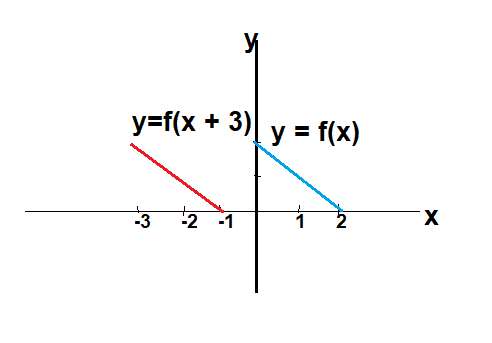# Transformation of Functions

I want to understand how the domain and range change upon applying transformations like (left/right shifts, up/down shifts, and vertical/horizontal stretching/compression) on functions.
Let f(x)=2-x if 0 ≤x ≤2 and 0 otherwise.
I want to describe the following functions 1) f(-x) 2) -f(x) 3) f(x+3) 4) f(x)+3 5) f(2x) 6) 2f(x) 7) f(2x+3)
The rule generates an output by multiplying the input by -1 and adding the result to 2.
1) f operates on the closed interval [0,2] as its domain. With f(-x) the domain then changes to [-2,0] so that upon multiplying each real number in this interval by -1 we obtain the same domain [0,2] and the same image [0,2] as f(x). f(-x)=2-(-x)=2+x.
2) -f(x) change the sign of the output keeping the input.
3) f(x+3) Each input in the closed interval [0,2] moves by 3 units, such that the domain changes to 3 ≤x+3 ≤5. However, under this domain the function generates an output of 0. As a result the domain of f(x+3) becomes [0,-1] so that upon adding 3 units to we wind up with the same domain and range as f(x).
4) Move every point up by 3 units
5) The domain of f(2x) shrinks to 0<x<1 so that when multiply each real number we obtain the same domain and range as f(x). f(2x)=2-2x
6) Multiply each output by 2 keeping the input.
7) Composed transformation of shrinking by 1/2 followed by a shift 3 units to the left resulting in a domain of [-3,-1].
In all of the above are we changing the rule f?

Homework Helper
I want to describe
You also want to learn how to post math using a little ##\ \LaTeX\ ##.
See tutorial. It's really easy, and it's fun. Enclose in ## for in-line math and in $$for displayed math. Example: Code: $$1) \quad  f(-x) \\ 2) \quad -f(x) \\ 3) \quad f(x+3) \\
4) \quad f(x)+3 \\5) \quad f(2x) \\6) \quad 2f(x) \\7) \quad f(2x+3)$$ yields$$1) \quad f(-x) \\ 2) \quad -f(x) \\ 3) \quad f(x+3) \\4) \quad f(x)+3 \\5) \quad f(2x) \\6) \quad 2f(x) \\7) \quad f(2x+3) this looks like ... because MathJax doesn't acknowledge the line feeds (\\\) and I don't understand what is screwing things up.A better way to do this is with \begin{align} and let ##\TeX## do the numbering: Code: \begin{align}
& f(-x)  \\  -&f(x) \\ &f(x+3) \\ &f(x)+3 \\&f(2x) \\2&f(x) \\ &f(2x+3)
\end{align}$$$$\begin{align}
& f(-x) \\ -&f(x) \\ &f(x+3) \\ &f(x)+3 \\&f(2x) \\2&f(x) \\ &f(2x+3)
\end{align}

##\ ##

Last edited:
Homework Helper
3) f(x+3) Each input in the closed interval [0,2] moves by 3 units, such that the domain changes to 3 ≤x+3 ≤5.
No. Make a sketch to see. You doubled up: domain is ##0 \le x+3 \le 2## .

In all of the above are we changing the rule f?
No, we are not.

##\ ##

No. Make a sketch to see. You doubled up: domain is
I reached the same answer but for the wrong reason.I thought that the x in f(x) is the same as x+3. Hence, why I added 3 to both sides of the inequality. So, we regard x+3 and x as just labels to the input?

Mentor
From post #1:
3) f(x+3) Each input in the closed interval [0,2] moves by 3 units, such that the domain changes to 3 ≤x+3 ≤5. However, under this domain the function generates an output of 0. As a result the domain of f(x+3) becomes [0,-1] so that upon adding 3 units to we wind up with the same domain and range as f(x).

No. Make a sketch to see. You doubled up: domain is ##0 \le x+3 \le 2## .
And further, ##0 \le x+3 \le 2 \Rightarrow -3 \le x \le -1##, so the domain for y = f(x + 3), is the translation left by 3 units of the domain for y = f(x).
I reached the same answer but for the wrong reason.I thought that the x in f(x) is the same as x+3.
You're dealing with two different functions, each of which is a translation by 3 units of the other.

Here's a sketch of y = f(x) (in blue) and y = f(x + 3) (in red).